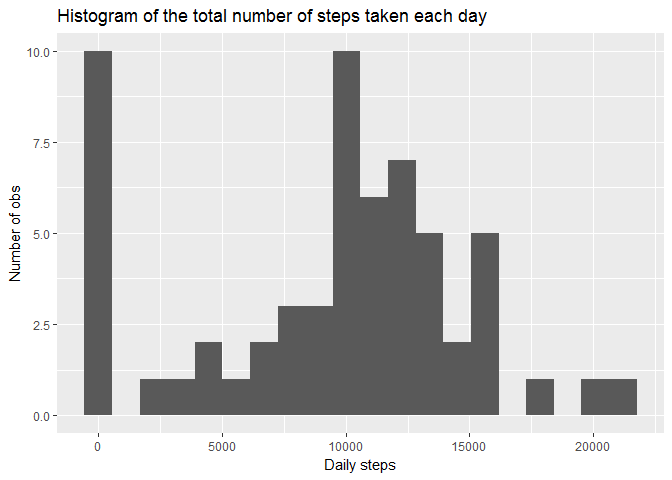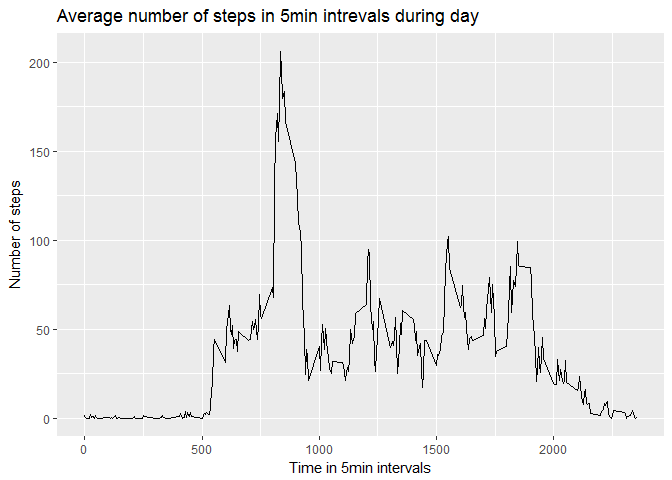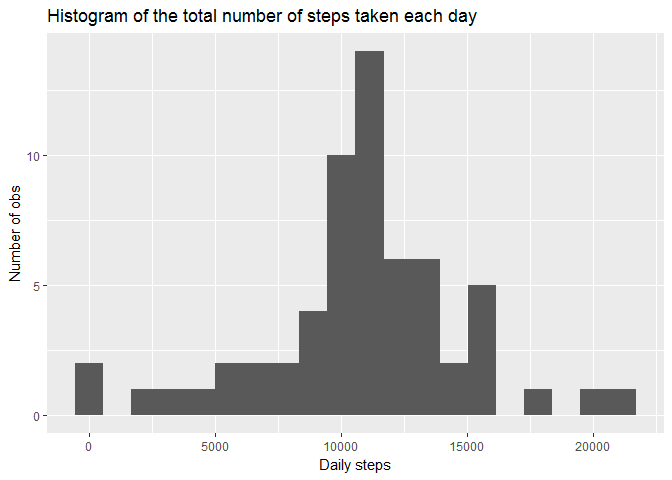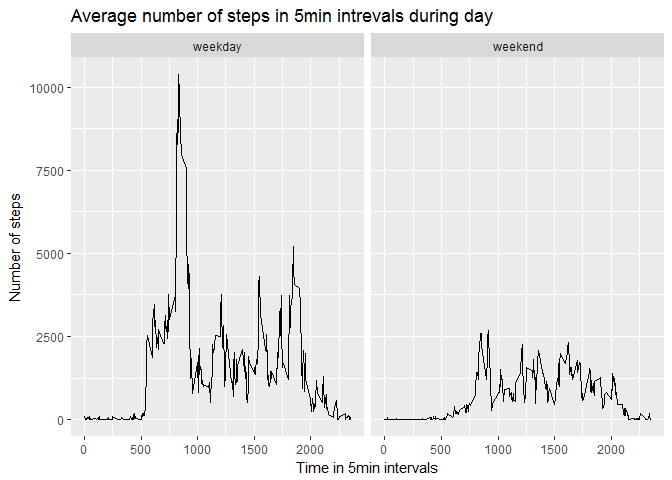# Get data from working directory
data <- read_csv("activity.zip", col_types = cols(date = col_datetime(format = "%Y-%m-%d")))

library(tidyverse)
library(lubridate)

Since data is stored in YYYY - MM - DD format, but time of day in a 5 min interval where 0 means midnight and for example 815 mean 8:15am i’m going to reformat it to proper time data.

data$date <- ymd(data$date)

# data$interval2 <- sprintf("%04d", data$interval)
# data$time<- format(strptime(data$interval2, format="%H%M"), format = "%H:%M")
# data$daytime <- paste(data$date, data$time, sep= " ") # data$daytime <- as.POSIXct(data$daytime, format="%Y-%m-%d %H:%M") ## What is mean total number of steps taken per day? The following histogram depicts the total number of steps each day: hist_data<- data %>% group_by(date) %>% summarise(daily_steps = sum(steps, na.rm=TRUE)) ggplot(hist_data, aes(x=daily_steps)) + geom_histogram(bins = 20) + labs(title="Histogram of the total number of steps taken each day", x = "Daily steps", y = "Number of obs")On average people where doing M = 9354.23 steps daily. Middle value of daily steps was Me = 1.039510^{4} # Mean daily steps: round(mean(hist_data$daily_steps, na.rm = TRUE), 2)
##  9354.23
# Median daily steps:
round(median(hist_data$daily_steps, na.rm = TRUE), 0) ##  10395 ## What is the average daily activity pattern? The following line graph depicts average steps of each 5min interval during all days of measurement: ts_data <- data %>% group_by(interval) %>% summarise(steps = mean(steps, na.rm=TRUE)) ggplot(ts_data, aes(x = interval, y= steps)) + geom_line() + labs(title="Average number of steps in 5min intrevals during day", x = "Time in 5min intervals", y= "Number of steps")data %>% group_by(interval) %>% summarise(steps = mean(steps, na.rm=TRUE)) %>% arrange(desc(steps)) %>% head(1) ## # A tibble: 1 x 2 ## interval steps ## <int> <dbl> ## 1 835 206.1698 On average 8:35 a.m. contais the maximum number of steps ## Imputing missing values There is a total of 2304 NA values in the steps column data %>% filter(is.na(steps)) %>% count() ## # A tibble: 1 x 1 ## n ## <int> ## 1 2304 In order to remove missing cases (NA values) I computed a 5min interval average across all days and replaced all NAs with it: data %>% group_by(interval) %>% mutate(mean_steps = mean(steps, na.rm = TRUE)) %>% ungroup() %>% select(steps, date, interval, mean_steps) ## # A tibble: 17,568 x 4 ## steps date interval mean_steps ## <int> <date> <int> <dbl> ## 1 NA 2012-10-01 0 1.7169811 ## 2 NA 2012-10-01 5 0.3396226 ## 3 NA 2012-10-01 10 0.1320755 ## 4 NA 2012-10-01 15 0.1509434 ## 5 NA 2012-10-01 20 0.0754717 ## 6 NA 2012-10-01 25 2.0943396 ## 7 NA 2012-10-01 30 0.5283019 ## 8 NA 2012-10-01 35 0.8679245 ## 9 NA 2012-10-01 40 0.0000000 ## 10 NA 2012-10-01 45 1.4716981 ## # ... with 17,558 more rows For future analysis I’ll keep a separate dataset where missing values have been replaced with computed averages dataNoNA <- data %>% group_by(interval) %>% mutate(steps = ifelse(is.na(steps), mean(steps, na.rm = TRUE), steps)) Above is a histogram of the total number of steps taken each day. hist_data2<- dataNoNA %>% group_by(date) %>% summarise(daily_steps = sum(steps, na.rm=TRUE)) ggplot(hist_data2, aes(x=daily_steps)) + geom_histogram(bins = 20) + labs(title="Histogram of the total number of steps taken each day", x = "Daily steps", y = "Number of obs")# Mean daily steps with NA's replaced: M<- round(mean(hist_data2$daily_steps, na.rm = TRUE), 2)
M
##  10766.19
# Median daily steps with NA's replaced:
Me<- round(median(hist_data2\$daily_steps, na.rm = TRUE), 0)
Me
##  10766

When NA values are replaced with 5min interval means for a given missing cell, the average of steps during a day is M = 1.07661910^{4}, and Median is Me = 1.076610^{4}

## Are there differences in activity patterns between weekdays and weekends?

dataNoNA <- dataNoNA %>%
mutate(weekday = if_else(weekdays(date) %in% c("sobota", "niedziela"),
"weekend", "weekday"))

Following is a panel plot containing a time series plot of the 5-minute interval (x-axis) and the average number of steps taken, averaged across all weekday days or weekend days (y-axis).

dataNoNA %>% group_by(weekday, interval) %>%
summarise(steps = sum(steps)) %>%
ggplot(aes(x = interval, y= steps)) +
geom_line() +
labs(title="Average number of steps in 5min intrevals during day",
x = "Time in 5min intervals", y= "Number of steps") +
facet_grid(.~weekday)